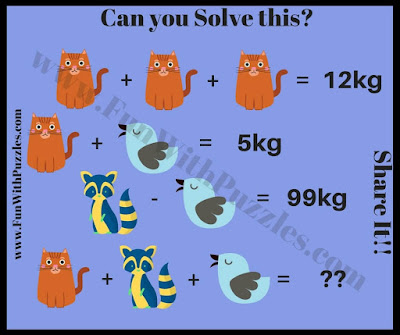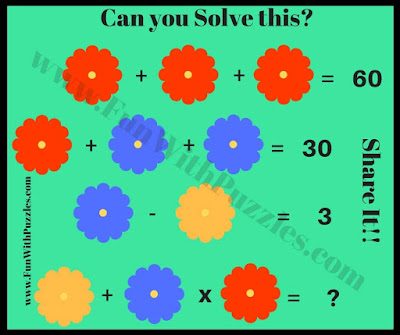Embark on a thrilling mathematical adventure as we present a trove of Mathematical Picture Puzzles meticulously designed for school students. The fusion of mathematics and visuals elevates the experience, transforming equations into interactive and enjoyable maths brain teasers.1. Shapes Picture Math Number Puzzle

Within these puzzles, the realm of mathematics comes to life through captivating visuals. As school students engage with the maths puzzles, they're tasked with solving equations using strategically placed objects within the picture. The challenge is both invigorating and enlightening, encouraging an interplay of observation and calculation.2. Flowers Picture Math Number Puzzle

While the puzzles exude an air of ease, their impact on math skills is profound. Students sharpen their logical thinking, nurture their attention to detail, and refine their problem-solving prowess. Each puzzle is a gateway to not just finding solutions but understanding the underlying mathematical concepts.3. Fruits Picture Math Number Puzzle

But the adventure doesn't end with solving—excitement awaits in the form of hidden answers. Clicking on the answer links unlocks the satisfaction of verification, creating a sense of accomplishment and curiosity.4. Fruits Picture Math Number Puzzle

Explore this assortment of puzzles, where math meets imagination, and equations unravel in captivating visuals. Beyond the challenge lies a journey of discovery, where mathematical prowess and creative thinking unite.

### Answers to 1 to 5th Math Brain Teasers6. Can you solve this Animal Picture Math Number Puzzle?7. Can you solve this Fun Picture Maths Number Puzzle?8. Solve this Fun Animals Picture Maths Number Puzzle9. Solve this Easy Picture Maths Number Brain Teaser10. Can you solve this Simple Picture Math Number Brain Teaser?

## List of Maths Puzzles and Brain Teasers

Don't forget to explore the following Maths Brain Teaser to twist your mind.

### Cool Maths Brain Teasers with Answers and Explanations

It contains mixed Maths Picture Puzzles in which one has to find the missing number among a group of a few numbers that share a logical and mathematical pattern with each other.

### Easy Mathematical Brain Teasers for Kids

It contains around 15 math puzzles with answers and explanations.

### Quick Brain Teasers with Answers

Here are five Fun Maths Picture Puzzles that can be solved very quickly.

Anthony TDK said...

Rajesh Kumar said...

I could not understand it. Can you please put more details about it?

Unknown said...

Unknown said...

Unknown said...

Plz explain

Unknown said...

14

Rajesh Kumar said...